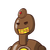# X can complete a piece of work in 30 h andY can complete it in 24 h. In how manyhours will it be completed if they wor

X can complete a piece of work in 30 h and
Y can complete it in 24 h. In how many
hours will it be completed if they work
together?​

### 1 thought on “X can complete a piece of work in 30 h and<br />Y can complete it in 24 h. In how many<br />hours will it be completed if they wor”

1.x can complete a work in 30hr

x’s work per hour = 1/30

Y completes a work in 24hr

So work done in one hour= 1/24

Now, work done by both in 1 hour

1/30 +1/24

= 4 + 5

120

= 9/120 = 3/40

Let z be the time taken to complete the work

therefore, 3/40 × z= 1

3z= 40

z= 40/3 = 13.33 hr